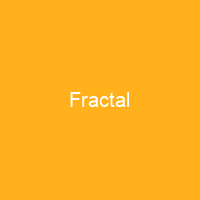# FractalIn mathematics, a fractal is a self-similar subset of Euclidean space. The term ‘fractal’ was first used by mathematician Benoit Mandelbrot in 1975. Analytically, fractals are usually nowhere differentiable. An infinite fractal curve can be conceived of as winding through space differently from an ordinary line.In mathematics, a fractal is a self-similar subset of Euclidean space whose fractal dimension strictly exceeds its topological dimension. The term ‘fractal’ was first used by mathematician Benoit Mandelbrot in 1975. Analytically, fractals are usually nowhere differentiable. An infinite fractal curve can be conceived of as winding through space differently from an ordinary line – although it is still 1-dimensional, its fractaldimension indicates that it also resembles a surface. Fractals are of particular relevance in the field of chaos theory, since the graphs of most chaotic processes are fractals. Many real and model networks have been found to have fractal features such as self similarity. The concept is difficult to define formally, even for mathematicians, but key features can be understood with a little mathematical background. The feature of’self-similarity’, for instance, is by analogy to zooming in with a lens or other device that zooms on finer, previously invisible, detail. If this is done on fractals, however, no new pattern appears; nothing changes and the same pattern repeats over and over. The difference for fractals is that the pattern must be detailed. A straight line is usually perceived as perceived as straight, for instance. This idea of being detailed relates to another feature that must be understood without much mathematical background: having a fractological dimension greater than itsTopological dimension, for example, refers to how a geometric shape is perceived compared to how it scales to how geometric shapes are usually perceived.